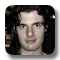# Optical Spring

## (A Processing program to show the effect of an optical spring)Alexandre Brieussel

2010

This Processing sketch illustrates the so-called `optical spring', a particular coupling between a mechanical oscillator and a light field. You can play with the parameters of the system and see how the laser field between the two mirrors couples the mechanical motion of these.

This applet has been built with Processing and makes use of the G4P (GUI for Processing) library and the gwoptics plotting library.

Light that sent to a mirror can be seen as tons of little specks hitting the surface of mirror. Each of those specks has a momentum. And when they are absorbed or reflected by the mirror, they transfer momentum to the mirror. We obtain a force, a so-called radiation pressure force, which we can employ to build an optical spring.

Light can also be described in the wave picture. If the length between two mirrors is an integer of two times the light wave length, these waves interfere constructively and you can obtain a power enhancement inside the cavity. We call this system a resonant Fabry Perot interferometer.

The applet above shows a case in which you need both features (particle and wave) of light to understand what is happening. The graph on the lower left shows the resonant enhancement of the light power inside the cavity, with the little box indicating the exact distance between the mirrors as develops in time. You can adjust the laser power, laser frequency and the average cavity length buttons to see what happens.

When you increase the power in the cavity, you increase the force acting on the mirrors, and the mirrors are pushed away from each other. Imagine that the mirrors are not moving and that the length between the mirrors correspond to a position on the right hand slope of the resonance peak. If the mirrors would now move a little bit further apart, as a result the power inside the cavity would decrease. Since the mirrors are suspended as pendulums, they would tend come back to their original position. If the mirrors are moving closer, the power in the cavity increases, and hence the radiation force increases. For little small movements, the system behaves exactly as if the two mirrors were connected by a spring. Just that this spring is not made of metal, but of photons.

http://www.physics.gla.ac.uk/igr/index.php?L1=members&L2=member&name=shild

Now if you excite the right hand mirror at one frequency, the other one will follow. And if excitation frequency is close to the resonance frequency of the spring, the left mirror, which has a smaller weight, will move with a much larger amplitude.

The applet was programmed during a summer project at the University of Glasgow, UK. See also our other Processing examples.